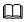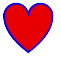path说明： 该组件从API version 7开始支持。后续版本如有新增内容，则采用上角标单独标记该内容的起始版本。

属性

id

string

-

d

string

-

• M/m = moveto
• L/l = lineto
• H/h = horizontal lineto
• V/v = vertical lineto
• C/c = curveto
• S/s = smooth curveto
• Q/q = quadratic Belzier curve
• T/t = smooth quadratic Belzier curveto
• A/a = elliptical Arc
• Z/z = closepath

示例

<!-- xxx.hml -->
<div class="container">
<svg width="400" height="400">
<path d="M 10,30 A 20,20 0,0,1 50,30 A 20,20 0,0,1 90,30 Q 90,60 50,90 Q 10,60 10,30 z"
stroke="blue" stroke-width="3" fill="red">
</path>
</svg>
</div>
1
2
3
4
5
6
7
8